## Tamilnadu Samacheer Kalvi 12th Chemistry Notes Chapter 7 Chemical Kinetics Notes

Chemical kinetics – The word kinetics is derived from the Greek word “kinesis” meaning movement. Chemical kinetics is the study of the rate and the mechanism of chemical reactions, proceeding under given conditions of temperature, pressure, concentration etc.

Rate of chemical reaction – A rate is a change in a particular variable per unit time. In a chemical reaction, the change in the concentration of the species involved in a chemical reaction per unit time gives the rate of a reaction.
Rate = $$\frac{-[\text { Change in the concentration of the reactants }]}{[\text { Change in time }]}$$

Unit of the rate of a reaction:
Unit of rate = $$\frac{\text { unit of concentration }}{\text { unit of time }}$$

Concentration is expressed in number of moles per litre and time is expressed in seconds and therefore the unit of the rate of a reaction is mol L-1s-1

Average rate – It is obtained by dividing the change in concentration of any of the reactant or product by the time taken for the change.

Instantaneous rate of a reaction – The rate of a reaction at a particular moment of time is called the Instantaneous rate of a reaction.

Rate law – The expression in which reaction rate is given in term of molar concentration of the reactants with each term raised to some power, which may or may not be same as the stoichiometric coefficient of the reacting species in a balanced chemical equation.
For a general reaction, xA+yB → products

The rate law for the above reaction is generally expressed as Rate = k[A]m [B]n
Where k is proportionality constant which is called rate constant. The values of m and n represent the reaction order with respect to A and B respectively.

Order of a reaction – The sum of exponents of the concentration of the reactants in the rate law expression.

Differences between rate and rate constant of a reaction

 Rate of a reaction Rate constant of a reaction 1. It represents the speed at which the reactants are converted into products at any instant. It is a proportionality constant 2. It is measured as decrease in the concentration of the reactants or increase in the concentration of products. It is equal to the rate of reaction, when the concentration of each of the reactants in unity 3. It depends on the initial concentration of reactants. It does not depend on the initial concentration of reactants.

Elementary reaction – Each and every single step in a reaction mechanism is called an elementary reaction.

Molecularity – The total number of reactant species that are involved in an elementary step is called molecularity of that particular step.

Differences between order and Molecularity

 Order of a reaction Molecularity of a reaction 1. It is the sum of the powers of concentration terms involved in the experimentally determined rate law. It is the total number of reactant species that are involved in an elementary step. 2. It can be zero (or) fractional (or) integer. It is always a whole number, cannot be zero or a fractional number. 3. It is assigned for a overall reaction. It is assigned for each elementary step of mechanism.

Examples for the first order reaction

(i) Decomposition of dinitrogen pentoxide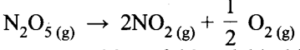(ii) Decomposition of thionylchloride;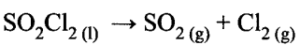(iii) Decomposition of the H202 in aqueous solution;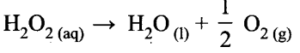(iv) Isomerisation of cyclopropane to propene.

Pseudo first order reaction – A second order reaction can be altered to a first order reaction by taking one of the reactant in large excess. Such reaction is called pseudo first order reaction.

Examples for a zero order reaction
(i) Photochemical reaction between H2 and Cl2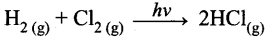(ii) Decomposition of N2O on hot platinum surface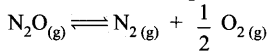(iii) lodination of acetone in acid medium is zero order with respect to iodine.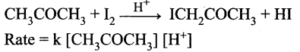Half life period of a reaction – The half life period of a reaction is defined as the time required for the reactant concentration to reach one half its initial value.

Collision theory – According to this theory, chemical reactions occur as a result of collisions between the reacting molecules.

Arrhenius equation

k= $$\mathrm{Ae}^{-\left(\frac{\mathrm{E}_{a}}{\mathrm{RT}}\right)}$$
A = Arrhenius factor (frequency factor)
R = Gas constant
k = Rate constant
Ea = Activation energy
T Absolute temperature (in K)

Factors affecting the reaction rate are,

1. Nature and state of the reactant
2. Concentration of the reactant
3. Surface area of the reactant
4. Temperature of the reaction
5. Presence of a catalyst

Samacheer Kalvi 12th Chemistry Notes